# MCQs on Electric Circuits

##### Page 61 of 63. Go to page 1 2 3 4 5 6 7 8 9 10 11 12 13 14 15 16 17 18 19 20 21 22 23 24 25 26 27 28 29 30 31 32 33 34 35 36 37 38 39 40 41 42 43 44 45 46 47 48 49 50 51 52 53 54 55 56 57 58 59 60 61 62 63
01․ The synchronous speed for the seventh space harmonic mmf wave of 8 pole, 50 Hz induction machine will be
5250 rpm in forward direction.
107.14 rpm in reverse direction.
107.14 rpm in forward direction.
5250 rpm in reverse direction.

Synchronous speed Ns = 120f /P = 120 X 50 / 8 = 750 rpm .MMF wave contains space harmonics eg. Fifth and seventh harmonics which corresponds to frequency five and seven times that of the fundamental. So seventh harmonic pole rotate forward at speed Ns /7 = 750/7= 107.14 rpm.

02․ Types of rotating machines
both B and C.
IM.
synchronous machine.
transformer.

Rotating machines are of 3 types - induction machine, synchronous machine and DC machine.

03․ Air gap flux density is of the order of
0.65 - 0.9 T.
0.75 - 0.8 T.
0.85 - 0.9 T.
0.35 - 0.5 T.

The air gap is present between stator and rotor. This air gap should be as low as possible. A reduced air gap will increase power factor. Hence air gap is should be low i.e., 0.35 - 0.5 gap flux density.

04․ A 100 ohm resistor is directly connected across a 80 V battery. What is the power dissipation?
64 W.
1 W.
10 W.
5 W.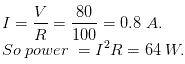05․ The number of electrons in the outer most orbits of carbon atom is
3.
4.
6.
7.

A carbon atom has 6 protons, there are 4 electrons orbiting in its outer shell and 2 within its inner shell.

06․ In a delta network each element has value R. The value of each element in equivalent star network will be
R / 6.
R / 4.
R / 2.
R / 3.

If the delta connected system has same resistance R at its three sides, then equivalent star resistance Req will be,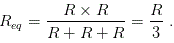07․ One atomic mass unit ( amu ) is approximately equal to
1.6 × 10-13 Kg.
1.6 × 10-24 Kg.
1.6 × 10-19 Kg.
1.6 × 10-27 Kg.

The atomic mass unit ( amu ) is approximately equal to the mass of one neutron or one proton.
1 amu = 1.66 × 10-27.

08․ If a large resistance is connected in parallel to a small resistance then net resistance will be
greater than the large resistance.
smaller than the small resistance.
smaller than the greater but larger than the smaller resistance.
none of the above option.

Numerically if we put any arbitrary values to find equivalent resistance, we get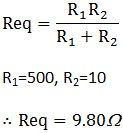But theoretically we know that there is current division in parallel circuit. So, to take more current the equivalent resistance must be low.(I = V/R). To increase I, the resistance R should be low.

09․ For the network shown in figure, the value of current through 8Ω resistor is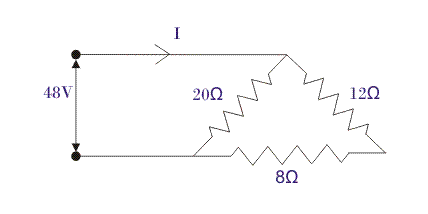4.8 A.
2.4 A.
1.5 A.
1.2 A.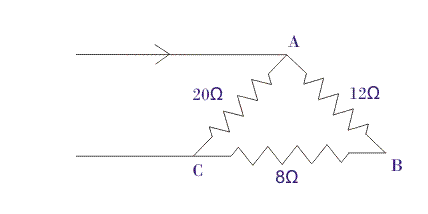There is no current division in the AB and BC branch. So, it is in series .The equivalent resistance is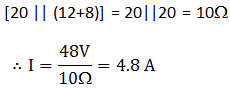Now, applying current division rule we get,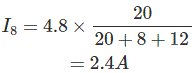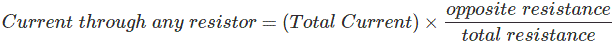10․ From the given figure find the R3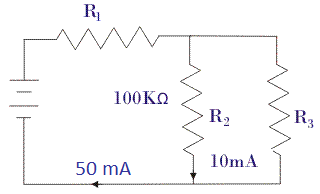25 mili ohm
25 ohm
25 kilo ohm
25 mega ohm

From the given figure we know the total current and the current through R2 resistance. So, we can find the R3 by using current division rule,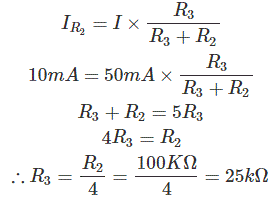<<<5960616263>>>